## Friday, 8 August 2014

### CHAPTER 11 - Worked Out Examples

Before we go on to solving examples involving the concepts we’ve seen till now, you are urged to once again go over the entire earlier discussion we’ve had, so that the “big picture” is clear in your mind.
 Example: 6
 Show that the vectors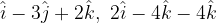$\hat i - 3\hat j + 2\hat k,\,\,2\hat i - 4\hat k - 4\hat k$ and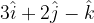$3\hat i + 2\hat j - \hat k$ are linearly independent.
 Solution: 6
Let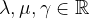$\lambda ,\mu ,\gamma \in\mathbb{R}$ be scalars such that$\lambda \left( {\hat i - 3\hat j + 2\hat k} \right) + \mu \left( {2\hat i - 4\hat j - 4\hat k} \right) + \gamma \left( {3\hat i + 2\hat j - \hat k} \right) = \vec 0$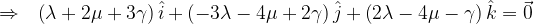$\Rightarrow \,\,\,\, \left( {\lambda + 2\mu + 3\gamma } \right)\hat i + \left( { - 3\lambda - 4\mu + 2\gamma } \right)\hat j + \left( {2\lambda - 4\mu - \gamma } \right)\hat k = \vec 0$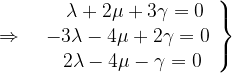$\Rightarrow \,\,\,\, \left. \begin{array}{l} \,\,\,\,\,\,\lambda + 2\mu + 3\gamma = 0\\ - 3\lambda - 4\mu + 2\gamma = 0\\ \,\,\,\,\,2\lambda - 4\mu - \gamma = 0 \end{array} \right\}$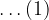$\ldots(1)$
The determined of the coefficient matrix is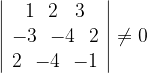$\left| {\begin{array}{*{20}{c}} 1\,\,\,\,2\,\,\,\,\,3\\ { - 3}\,\,\,\,{ - 4}\,\,\,\,2\\ 2\,\,\,\,{ - 4}\,\,\,\,{ - 1} \end{array}} \right| \ne 0$
Thus, the system of equations in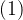$(1)$ has no solution for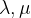$\lambda ,\mu$ and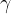$\gamma$ apart from the trivial solution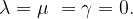$\lambda = \mu \,\, = \gamma = 0.$ This implies that the three vectors are linearly independent.

#### COLLINEARITY OF POINTS

 Example: 7
 Let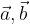$\vec a,\vec b$ and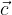$\vec c$ be three non-coplanar vectors. Prove that the points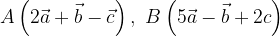$A\left( {2\vec a + \vec b - \vec c} \right),\,\,B\left( {5\vec a - \vec b + 2c} \right)$ and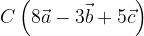$C\left( {8\vec a - 3\vec b + 5\vec c} \right)$ are collinear. When we say the point$P\left( {\vec p} \right)$, we mean the point whose position vector, i.e, the vector drawn from the origin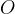$O$ to that point,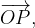$\overrightarrow {OP} ,$ is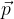$\vec p$.
 Solution: 7
We have been given the position vectors of three points and we are required to prove that they are collinear. Let us see what condition must be satisfied in order for three points to be collinear:Thus, there must be some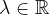$\lambda \in \mathbb{R}$ for which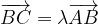$\overrightarrow {BC} = \lambda \overrightarrow {AB}$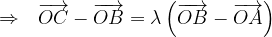$\Rightarrow \,\,\,\, \overrightarrow {OC} - \overrightarrow {OB} = \lambda \left( {\overrightarrow {OB} - \overrightarrow {OA} } \right)$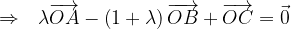$\Rightarrow \,\,\,\, \lambda \overrightarrow {OA} - \left( {1 + \lambda } \right)\overrightarrow {OB} + \overrightarrow {OC} = \vec 0$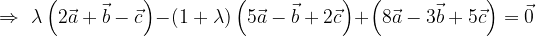$\Rightarrow \,\, \lambda \left( {2\vec a + \vec b - \vec c} \right) - \left( {1 + \lambda } \right)\left( {5\vec a - \vec b + 2\vec c} \right) + \left( {8\vec a - 3\vec b + 5\vec c} \right)=\vec 0$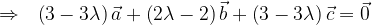$\Rightarrow \,\,\,\, \left( {3 - 3\lambda } \right)\vec a + \left( {2\lambda - 2} \right)\vec b + \left( {3 - 3\lambda } \right)\vec c = \vec 0$
Since$\vec a,\vec b$ and$\vec c$ are non-coplanar, we have$3 - 3\lambda = 0$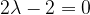$2\lambda - 2 = 0$$3 - 3\lambda = 0$
This consistently gives the solution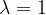$\lambda = 1$, implying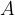$A$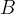$B$ and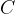$C$ are collinear.